# An Introduction to ARM NEON

## 03 Jan 2014

Categories: Code Tags: ARM NEON Assembly Windows Phone 8

NEON is ARM’s take on a single instruction multiple data (SIMD) engine. As it becomes increasingly ubiquitous in even low-cost mobile devices, it is more worthwhile than ever for developers to take advantage of it where they can. NEON can be used to dramatically speed up certain mathematical operations and is particularly useful in DSP and image processing tasks.

In this post I will show how it can improve a simple image processing algorithm and will compare it to several other approaches. My particular implementation will be written for Windows Phone 8, however the principles should be platform agnostic and therefore easily applicable to Android and iOS as well.

### Testbed application: Sepiagram

We will use NEON to speed up an exciting image processing task: applying sepia tone to a photograph. The basic algorithm given in this blog post will serve as a starting point. Output RGB values are a simple linear combination of input RGB values, with red and green given higher weights than blue in order to give the image a yellowish hue:

``````outputRed = (inputRed * 0.393) + (inputGreen * 0.769) + (inputBlue * 0.189)
outputGreen = (inputRed * 0.349) + (inputGreen * 0.686) + (inputBlue * 0.168)
outputBlue = (inputRed * 0.272) + (inputGreen * 0.534) + (inputBlue * 0.131)
``````

The app skeleton is as equally simple, consisting of an image view, image chooser, and several buttons for applying our various implementations of the sepia tone algorithm. I’ve also included some timing code that allows us to very roughly compare the performance of each approach.

### Naive C# implementation

Our first implementation in C# is as straightforward as one might expect:

``````public static void ApplySepia(WriteableBitmap Bitmap)
{
for (var i = 0; i < Bitmap.Pixels.Length; ++i)
{
var pixel = Bitmap.Pixels[i];

// Extract red, green, blue components.
var ir = (pixel & 0xFF0000) >> 16;
var ig = (pixel & 0xFF00) >> 8;
var ib = pixel & 0xFF;

// Apply the transformation.
var or = (uint)(ir * 0.393f + ig * 0.769f + ib * 0.189f);
var og = (uint)(ir * 0.349f + ig * 0.686f + ib * 0.168f);
var ob = (uint)(ir * 0.272f + ig * 0.534f + ib * 0.131f);

// Saturate the result.
or = or > 255 ? 255 : or;
og = og > 255 ? 255 : og;
ob = ob > 255 ? 255 : ob;

// Write the resulting pixel back to the bitmap.
Bitmap.Pixels[i] = (int)(0xFF000000 | or << 16 | og << 8 | ob);
}
}
``````

The only thing that might be immediately non-obvious is how we must saturate the output results. As we’re working with 32-bit integers and the color weights total to more than 1, it’s possible for the output values to be greater than 255. We therefore cap (saturate) them to 255.

On my Lumia 1020 this implementation takes around 600-800ms for a 3072x1728 pixel image. Not bad, but we can do much better.

### Naive to native: C++

It turns out that floating point operations are slow1 and an immediate improvement can be made by modifying the algorithm slightly to process integers instead of floats. We can scale up each color weight by some factor, apply the transformations, then scale the results back down. As our RGB values are integers between 0 and 255 anyway, this completely eliminates any need for floating point arithmetic. We will choose 1024 as our scale factor as the color weights are given to 3 decimal places (so we lose no precision) and it is a convenient power-of-2. Hence, we can rewrite the equations as:

``````outputRed = ((inputRed * 402) + (inputGreen * 787) + (inputBlue * 194)) / 1024
outputGreen = ((inputRed * 357) + (inputGreen * 702) + (inputBlue * 172)) / 1024
outputBlue = ((inputRed * 279) + (inputGreen * 547) + (inputBlue * 134)) / 1024
``````

In C++, this becomes:

``````void NEONRT::NEONRT::IntegerSepia(const Platform::Array^ image)
{
uint32_t *px = (uint32_t *)image->Data;
uint32_t *end = px + image->Length;

for (; px < end; ++px) {
// Extract red, green, blue components.
unsigned int ir = (*px & 0x00FF0000) >> 16;
unsigned int ig = (*px & 0x0000FF00) >> 8;
unsigned int ib = (*px & 0x000000FF);

// Apply the transformation.
unsigned int or = (ir * 402 + ig * 787 + ib * 194) >> 10;
unsigned int og = (ir * 357 + ig * 702 + ib * 172) >> 10;
unsigned int ob = (ir * 279 + ig * 547 + ib * 134) >> 10;

// Saturate the result.
or = or > 255 ? 255 : or;
og = og > 255 ? 255 : og;
ob = ob > 255 ? 255 : ob;

// Write the resulting pixel back to the bitmap.
*px = 0xFF000000 | (unsigned int)or << 16 | (unsigned int)og << 8 | (unsigned int)ob;
}
}
``````

Despite the fact we have 3 more divisions per pixel in this version than the original, this implementation takes around 300-400ms on the same image – already a 2x speed increase.

### Utilizing NEON

To briefly recap: NEON is a single instruction multiple data (SIMD) architecture meaning it can perform the same arithmetic operation on multiple data values in parallel. It has 32x 64-bit registers, named `d0-d31` (which can also be viewed as 16x 128-bit registers, `q0-q15`)2. These registers are considered as vectors of elements of the same data type. NEON arithmetic instructions typically distinguish between these data types in order to apply the same operation to all lanes. For example, `vadd.f32` considers a 64-bit register as 2x 32-bit floats, whereas `vadd.i8` considers it as 8x 8-bit signed integers. For a more substantive description, please see the official ARM documentation.

Back to our sepia color transformation equations.

On a conventional single instruction single data machine, the sepia algorithm requires a total of 9 multiplications and 6 additions per pixel. Using NEON SIMD instructions, however, we can operate on vectors all in one go. We can rewrite the above formula in terms of vector multiplications and additions:

``````[outputRed  outputGreen  outputBlue] = inputRed   * [0.393  0.349  0.272]
+ inputGreen * [0.769  0.686  0.534]
+ inputBlue  * [0.189  0.168  0.131]
``````

This requires only 3 vector multiplications and 2 vector additions per pixel. In fact, NEON includes a vector multiply and accumulate instruction which simultaneously performs a vector multiplication and addition. Using 1 multiply and 2 multiply-accumulates, we can reduce the total number of operations to 3.

We will walk through one iteration of a loop that processes multiple pixels at a time. We have a 32-byte chunk of memory containing 8x 32-bit pixels. These pixels are further subdivided into 4x 8-bit subpixels – alpha, red, green and blue (ARGB). Each block in the figure below represents 1 byte: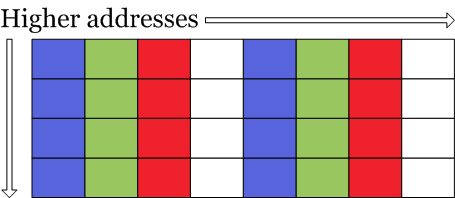NEON allows us to load 32 bytes using a single opcode:

``````vld1.u32 { d20, d21, d22, d23 }, [r0]
``````

This has the effect of loading 2 pixels into each of the registers `d20-d23`. Register `r0` is a pointer to the 8-pixel block of memory within the bitmap. Now we have: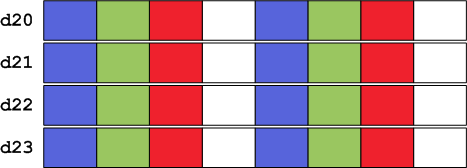It should be immediately obvious that we cannot simply multiply in-place by our weights as each subpixel’s value will probably overflow past 255. Therefore we must extend each subpixel to 16 bits:

``````vmovl.u8 q0, d20
vmovl.u8 q1, d21
vmovl.u8 q2, d22
vmovl.u8 q3, d23
``````

Note the `.u8` suffix on these instructions tell NEON to treat the input registers (`d20-d23` in this case) as vectors of 8-bit values (i.e. the subpixels). This is an important distinction as our output would be structured differently if, for example, we used the similar `vmovl.u16` instruction3. Now the two pixels contained in 64-bit-wide `d20` are extended and copied to the 128-bit-wide `q0`, and similarly for `d21-d23` and `q1-q3`. Lets now consider only the first pixel, which is contained in register `d0`: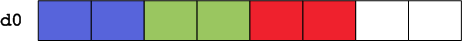Again, 2 bytes per subpixel is not enough to guarantee we won’t overflow: considering that we are multiplying each subpixel (max 2^8) by its combined weights (> 1) and also by 1024 (2^10), we need at least 18 bits to represent the intermediate values before we again divide by 1024. Luckily, NEON provides vector multiply and multiply-accumulate instructions that automatically widen their outputs. We therefore perform our three vector arithmetic operations, starting with a multiply:

``````vmull.u16 q4, d16, d0
``````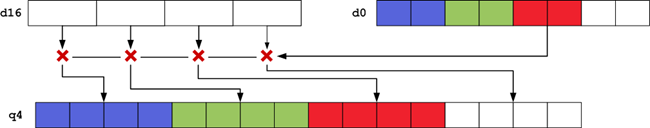Here `q4` is the destination register, `d16` contains our red color weights and `d0` contains the pixel we are currently operating on. Note that this form of the `vmul` instruction takes a 16-bit scalar as its 3rd argument and hence we select the red subpixel by subscripting `d0`. Next, we multiply by the green weights, contained in `d17`, and accumulate into the destination register:

``````vmlal.u16 q4, d17, d0
``````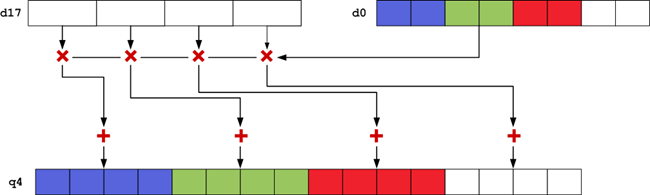And then similarly for the blue weights, contained in `d18`:

``````vmlal.u16 q4, d18, d0
``````

After repeating for the other 7 pixels (using a unique `q` register for each, we have 16 after all!), we then perform a right shift by 10 to divide by 1024, narrow and saturate:

``````vqshrn.u32 d0, q4, 10
/* and the rest ... */
``````

Here `d0` is the destination register, `q4` still contains our first pixel, and 10 is a constant. This is the saturating form of the shift-right instruction meaning it will cap output values to 2^16 - 1 if they would otherwise overflow their smaller destinations. After repeating another 7 times, we perform the final saturating narrow to fit our pixels back into 4-bytes:

``````vqmovn.u16 d0, q0
/* and the rest ... */
``````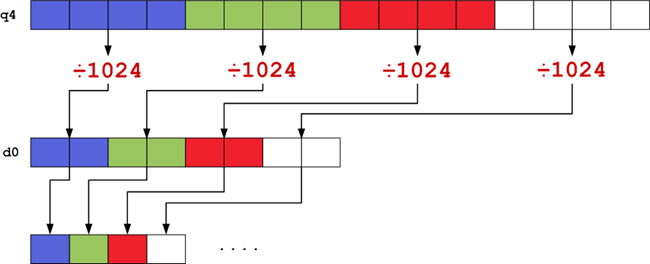Finally, we set the alpha value to 255 using a bitwise OR and write the results back out to the bitmap:

``````vorr d0, d0, d19
/* ... */
vst1.32 { d0, d1, d2, d3 }, [r0]
``````

Here `d0-d3` contain our 8 pixels, `d19` contains the constant `0xFF000000FF000000`, and `r0` is still a pointer to the 8-pixel block in the bitmap.

### What else?

The rest of the assembly routine is mainly concerned with setting up the registers containing the color weights, and looping. A link to the full source code of the app can be found at the end of this post.

### Results

The resulting NEON implementation takes roughly 80-100ms – an impressive difference, considering the assembly routine is probably not particularly optimized.

To summarize, the results on my Lumia 1020 for each implementation are as follows:

Implementation Time/ms (average of 10 runs)
C# (floating point) 697
C++ (integer) 228
NEON 94

### Why not NEON intrinsics?

NEON intrinsics are C/C++ macros that compile down to single NEON instructions, at least in theory. In practice I have found that modern compilers still produce downright awful NEON code. My first implementation of the sepia algorithm using NEON intrinsics in C++ performed worse than the naive C# implementation. Looking at the generated assembly, the compiler seems to love pointlessly4 spilling registers into RAM which drastically reduces performance. Thinking that the compiler simply wasn’t intelligent enough to unroll each loop, I manually unrolled them, keeping the theoretical effect of the code exactly the same. The algorithm then did run much faster, but also completely incorrectly, producing vertical blue lines in the output. I didn’t have the time or will to work out why, but either it’s a bug in the Visual C++ compiler or I’m breaking some rules somewhere that I don’t yet know about.

### Final thoughts

• NEON can dramatically improve performance of algorithms that can take advantage of data parallelism.
• Compiler support for NEON is still terrible.
• It’s not much more difficult to write assembly than struggle with NEON instrinsics. You can beat the compiler.
• It’s can be worth converting your algorithm to avoid floating point operations1.
• Don’t make any conclusions about performance without thorough benchmarking.

### Source code

1. Not really true in general. Whether floating point operations are slower than integer operations depends on a vast number of factors. From what I’ve experienced on ARM, however, it is often the case. ↩︎

2. NEON actually shares its registers with the floating point unit, if one exists. ↩︎

3. `vmovl.u8` will transform `0xFFFFFFFF` to `0x00FF00FF00FF00FF`, whereas `vmovl.u16` will transform it to `0x0000FFFF0000FFFF`↩︎

4. There may be a good reason I don’t know about, such as certain registers being expected to be preserved across function calls. I’d have thought the compiler would deal with this, however… ↩︎